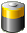# PID Controller

## CONTROLLER PROPERTIES

The PID-Controller is a combination of a proportional, derivative and integral controller.

The proportional term produces an output value that is proportional to the current error value. The derivative of the process error is calculated by determining the slope of the error over time and multiplying this rate of change by the derivative term. The contribution from the integral term is proportional to both the magnitude of the error and the duration of the error.

The idea is that you first set Ki value to zero and increase Kp until the signal oscillates then set the value of Kp to roughly half. Then increase Ki until any offset is corrected quickly enough for the process. Finally increase Kd if needed to ensure the controller is quick enough to reach the target value after a disturbance.

## OVERVIEW

#### INPUTS

 AI Actual Valve Connect the analogue input here A Automatic input 1 = Automatic 0 = Manual (Mv value is output at AQ) R Reset input A pulse at R causes the controller to start regulating at 0

#### PARAMETERSRemanence Sets the function block to be remanent, e.g. return to the last known scene after a power cut T Target value The desired setpoint for the controller to reach ST Sampling time Time interval for the new output value to be computed Tr Threshold If error is less than the threshold parameter AQ will remain the same Kp Proportional term Causes an output value that is proportional to the current error value Ki Integral term Is proportional to both the magnitude of the error and the duration of the error Kd Derivative term Calculates the derivative of the process error Mv Analogue value for manual mode This parameter is output to AQ if manual mode is used (A = 0) Min Minimum value Minimum output value of AQ Max Maximum value Maximum output value of AQ

#### OUTPUTS

 AQ Analogue output The controlled signal is output here